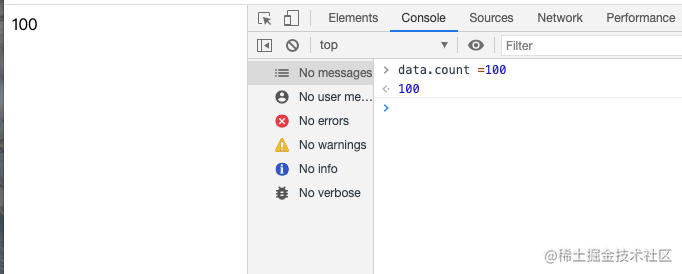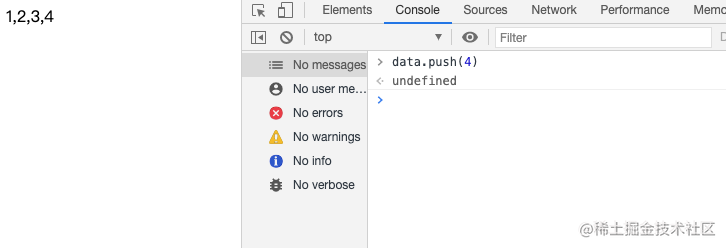# 浅谈Vue响应式原理

Vue.js 的核心包括一套“响应式系统”。

“响应式”，是指当数据改变后，Vue 会通知到使用该数据的代码。例如，视图渲染中使用了数据，数据改变后，视图也会自动更新。

Vue中响应式数据分为:对象类型`{}`和数组类型`[]`

## 对象类型 {}

• 数据`data`
• 数据监听器`defineReactive`
• 订阅者（更新视图）`watcher`
• 维护订阅者`dep`
• 状态`active`

``````    <div id="app"></div>

<script>
let data = {
count:0
}
app.innerHTML = data.count
</script>

### 1.添加视图更新watcher

``````    <div id="app"></div>
<script>
let data = {
count:0
}
// 定义watcher函数，传入参数为函数，且立即执行
let watcher = (fn)=>{
fn();
}

watcher(()=>{
// 更换app内容
app.innerHTML = data.count;
})
</script>

`watcher`所执行的操作 将页面上的内容更新=>视图更新

### 2.实现数据监听器

``````    <script>
let data = {
count:0
}
// 数据监听器
function defineReactive(obj) {
//每一个属性都重新定义get、set
for(let key in obj){
let value = obj[key]
Object.defineProperty(obj,key,{
// 当data中的值“出现”的时候，执行get（）
get(){
//将获取的原始值返回
return value;
},
// 当data中的值“改变”的时候，执行get（）
set(newValue){
value = newValue
}
})
}
}
//劫持data中的数据
defineReactive(data)

//此时的a没有被数据监听器监测到，属于“后来者”不受劫持
data.a = 10;

// 定义watcher函数，传入参数为函数，且立即执行
let watcher = (fn)=>{
fn();
}

watcher(()=>{
// 取值
app.innerHTML = data.count;
})
</script>

### 3. 当数据改变时更新视图

``````   <div id="app"></div>
<script>
let data = {
count:0
}
//需要执行的视图内容
let active;
// 数据监听器
function defineReactive(obj) {
for(let key in obj){
let value = obj[key];
//存放当前属性相关的所有方法
let dep = [];
Object.defineProperty(obj,key,{
// 当data中的值“出现”的时候，执行get（）
get(){
//(3)
if(active){
dep.push(active)
}
return value
},
// 当data中的值“改变”的时候，执行get（）
set(newValue){
value = newValue
dep.forEach(active=>active())
}
})
}
}
//劫持data中的数据
defineReactive(data)

// 定义watcher函数，传入参数为函数，且立即执行
let watcher = (fn)=>{
active = fn;
//(1)
fn();
//(4)
active = null;
}

watcher(()=>{
// 更换app内容
//(2)
app.innerHTML = data.count;
})
</script>Vue2.0响应式用的是`Object.defineProperty` Vue3.0响应式用的是`proxy`

`data`中的数据存在多层嵌套的时候，如果用`Object.defineProperty`,内部会进行递归，影响性能。`proxy`提升性能，但是不兼容ie11。

## 数组类型 []

``````    <div id="app"></div>
<script>
let data = [1,2,3];
// 获取数组所有方法-原型链
let originArray = Array.prototype;
// 浅拷贝
let arrayMethods = Object.create(originArray);
// 数据监听器
function defineReactive(obj) {
// 函数劫持，重写方法。可以添加自己想要执行的内容
arrayMethods.push = function (...args) {
// 更改this指向
originArray.push.call(this,...args);
render();
}
// 挂载到原型上
obj.__proto__ = arrayMethods
}
defineReactive(data)

// 视图渲染
function render() {
app.innerHTML = data;
}
render();

</script>